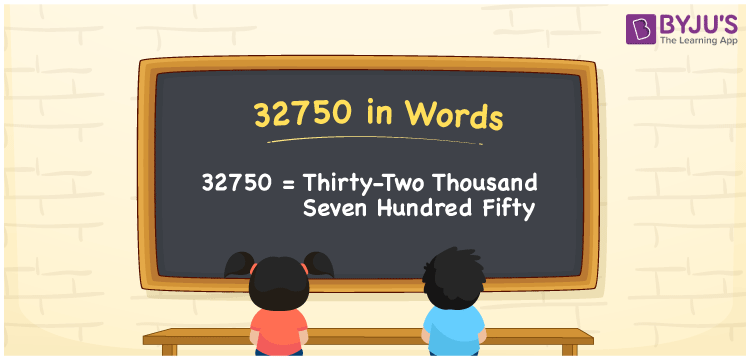# 32750 in words

32750 in words is written as Thirty Two Thousand Seven Hundred and Fifty. In 32750, 3 has a place value of ten thousand, 2 is in the place value of thousand, 7 is in the place value of hundred and 5 is in the place value of ten. The article on Place Value gives more information. The number 32750 is used in expressions that relate to money, distance, social media views, and many more. For example, “The postal code in Florida, USA, is Thirty Two Thousand Seven Hundred and Fifty.”

 32750 in words Thirty Two Thousand Seven Hundred and Fifty Thirty Two Thousand Seven Hundred and Fifty in Numbers 32750

## 32750 in English Words## How to Write 32750 in Words?

We can convert 32750 to words using a place value chart. The number 32750 has 5 digits, so let’s make a chart that shows the place value up to 5 digits.

 Ten thousand Thousands Hundreds Tens Ones 3 2 7 5 0

Thus, we can write the expanded form as:

3 × Ten thousand + 2 × Thousand + 7 × Hundred + 5 × Ten + 0 × One

= 3 × 10000 + 2 × 1000 + 7 × 100 + 5 × 10 + 0 × 1

= 32750.

= Thirty Two Thousand Seven Hundred and Fifty.

32750 is the natural number that is succeeded by 32749 and preceded by 32751.

32750 in words – Thirty Two Thousand Seven Hundred and Fifty.

Is 32750 an odd number? – No.

Is 32750 an even number? – Yes.

Is 32750 a perfect square number? – No.

Is 32750 a perfect cube number? – No.

Is 32750 a prime number? – No.

Is 32750 a composite number? – Yes.

## Solved Example

1. Write the number 32750 in expanded form

Solution: 3 x 10000 + 2 x 1000 + 7 x 100 + 5 x 10 + 0 x 1

Or Just 3 x 10000 + 2 x 1000 + 7 x 100 + 5 x 10

We can write 32750 = 30000 + 2000 + 700 + 50 + 0

= 3 x 10000 + 2 x 1000 + 7 x 100 + 5 x 10 + 0 x 1.

## Frequently Asked Questions on 32750 in words

Q1

### How to write the number 32750 in words?

32750 in words is written as Thirty Two Thousand Seven Hundred and Fifty.
Q2

### State whether True or False. 32750 is divisible by 3?

False. 32750 is not divisible by 3.
Q3

### Is 32750 divisible by 10?

Yes. 32750 is divisible by 10.